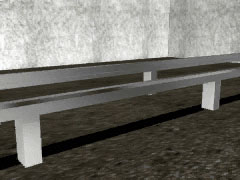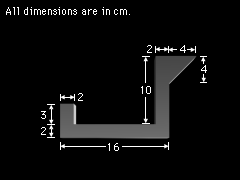Ch 7. Centroid/Distributed Loads/Inertia Multimedia Engineering Statics Centroid: Line Area Vol Centroid: Composite Distributed Loads Area Moment of Inertia
 Chapter 1. Basics 2. Vectors 3. Forces 4. Moments 5. Rigid Bodies 6. Structures 7. Centroids/Inertia 8. Internal Loads 9. Friction 10. Work & Energy Appendix Basic Math Units Sections Search eBooks Dynamics Fluids Math Mechanics Statics Thermodynamics Author(s): Kurt Gramoll ©Kurt GramollSTATICS - CASE STUDY IntroductionProblem Description A luggage transport system for a new airport is having some problems. The railway track bends when the luggage carrier passes over it and will probably break. To analyze this bending, engineers must determine the neutral axis of the beam, which for a homogeneous beam passes through the centroid of the cross section. (The area moment of inertia of the cross section will be found in a latter section). What is known: The dimensions of the cross section are as shown. QuestionRail Cross Section Where is the centroid of the cross section? Approach Break the beam cross section into simpler, component parts. Determine the centroid and area of each component part. Use the method of composite parts to calculate the x and y locations of the centroid of the cross section.

Practice Homework and Test problems now available in the 'Eng Statics' mobile app
Includes over 500 problems with complete detailed solutions.
Available now at the Google Play Store and Apple App Store.